# Introduction to LaTeX

Mar. 4, 2015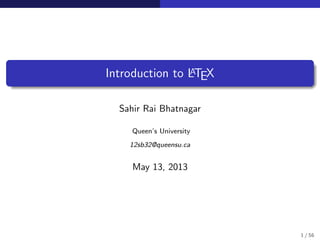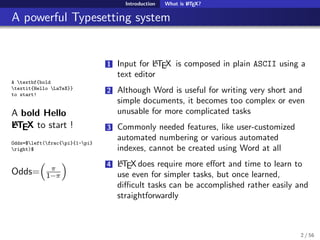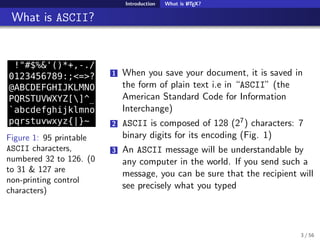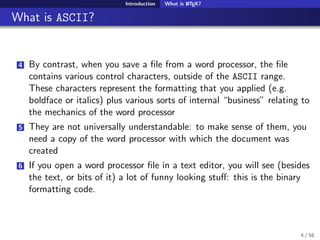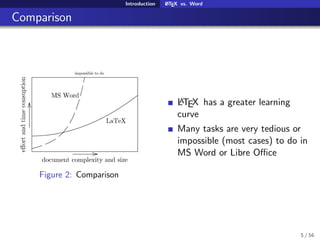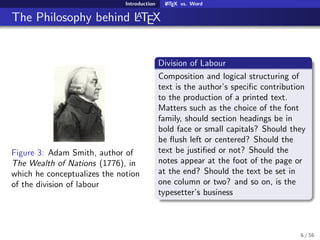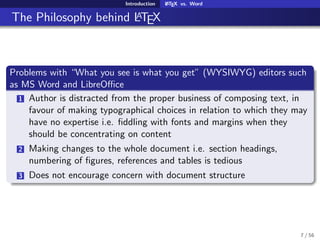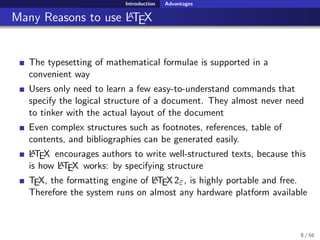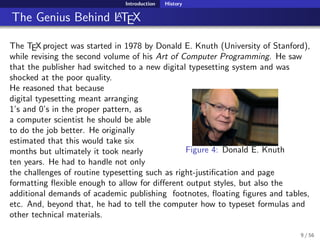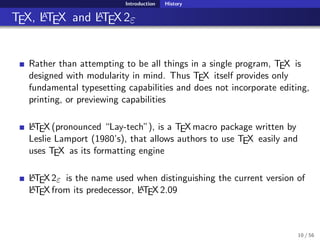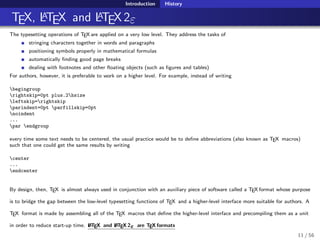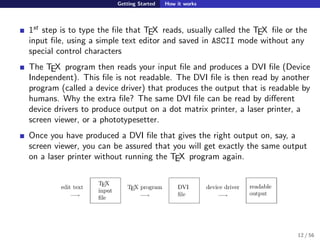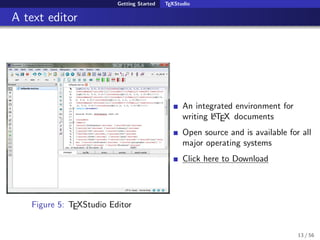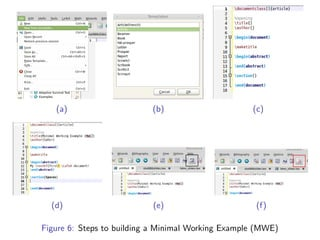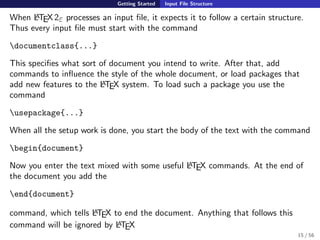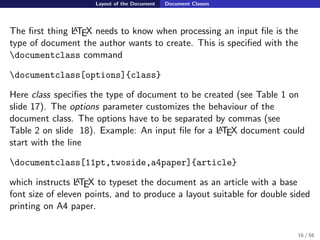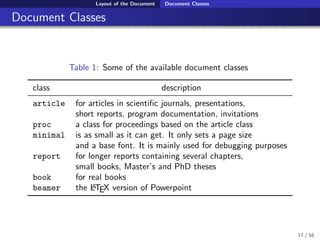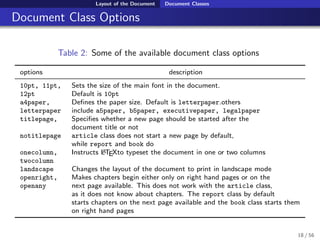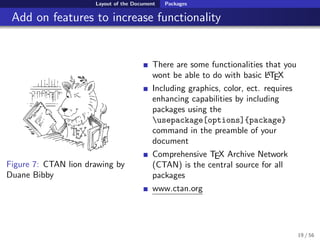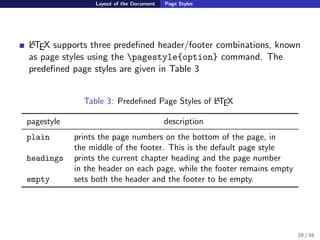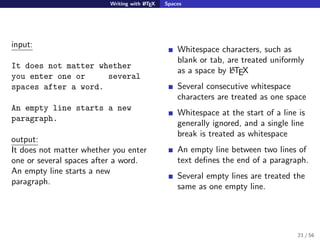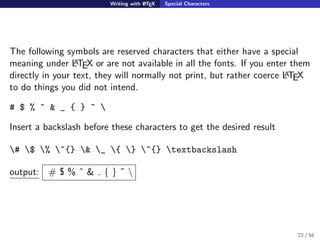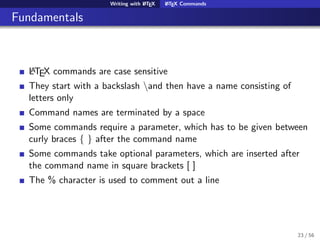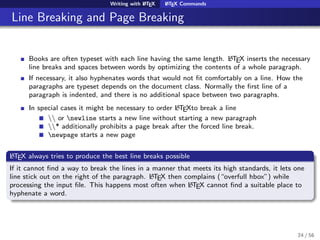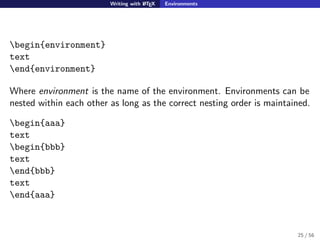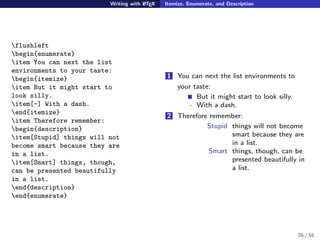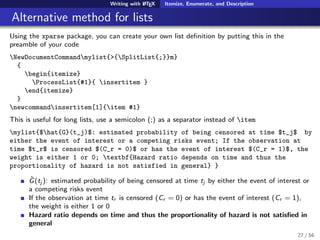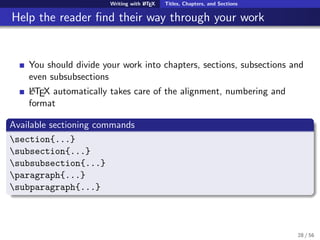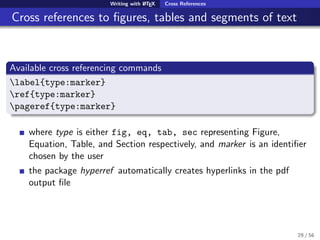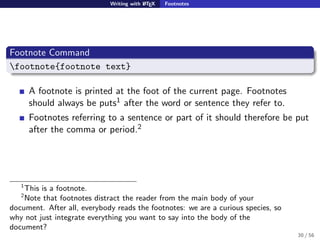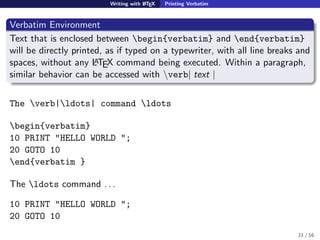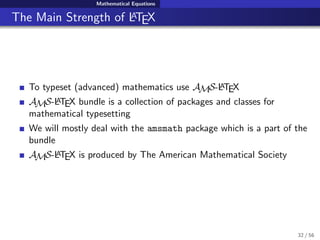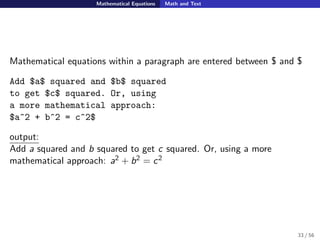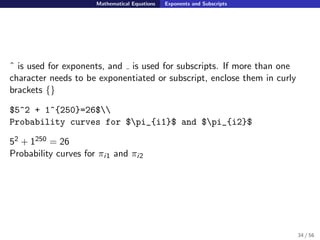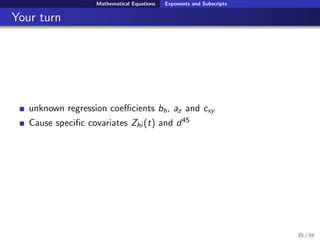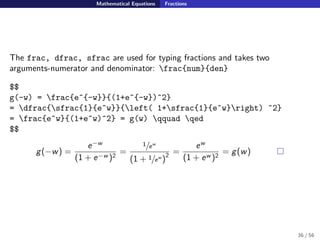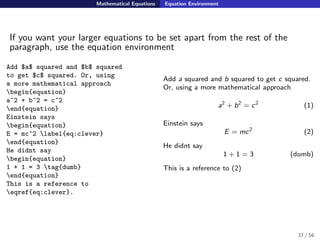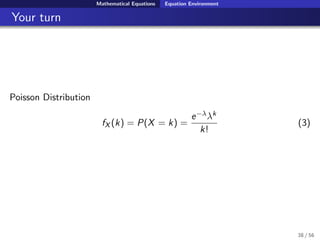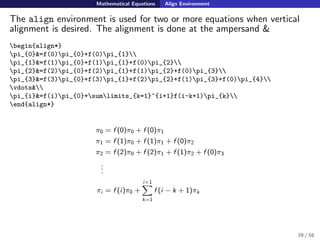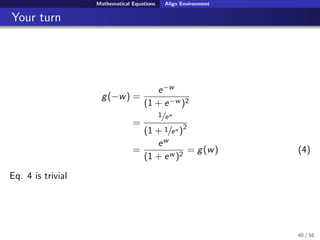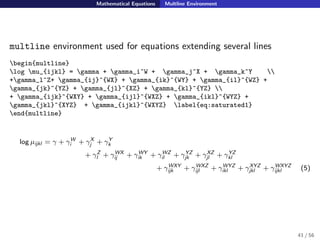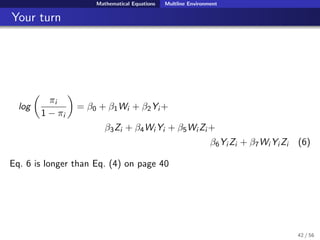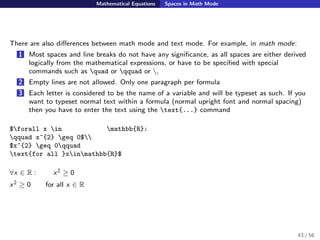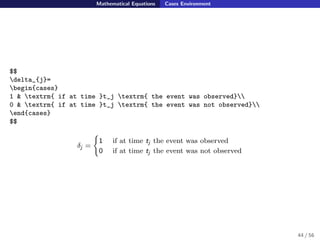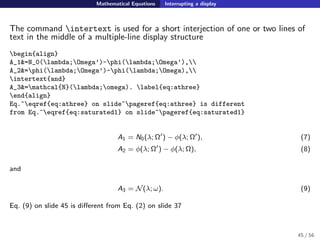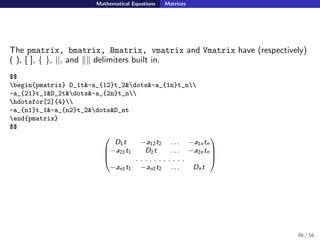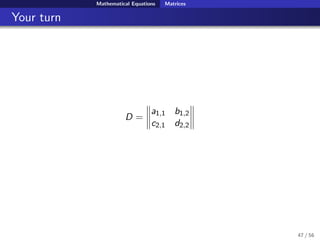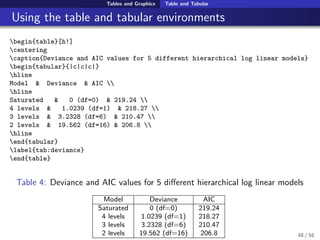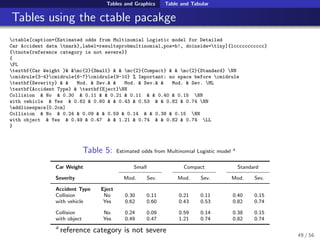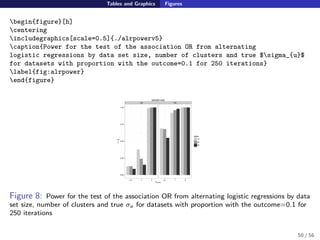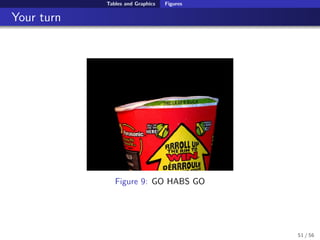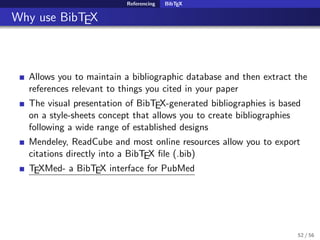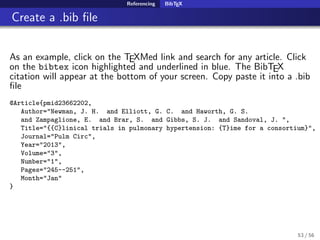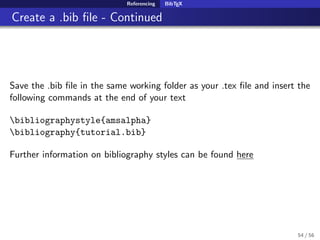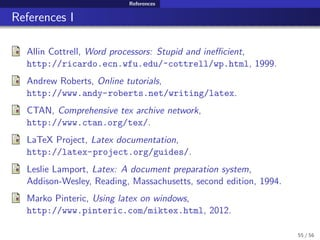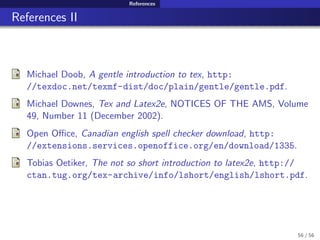1 of 56

### Introduction to LaTeX

1. Introduction to LATEX Sahir Rai Bhatnagar Queen’s University 12sb32@queensu.ca May 13, 2013 1 / 56
2. Introduction What is LATEX? A powerful Typesetting system A textbf{bold textit{Hello LaTeX}} to start! A bold Hello LATEX to start ! Odds=$left(frac{pi}{1-pi} right)$ Odds= π 1−π 1 Input for LATEX is composed in plain ASCII using a text editor 2 Although Word is useful for writing very short and simple documents, it becomes too complex or even unusable for more complicated tasks 3 Commonly needed features, like user-customized automated numbering or various automated indexes, cannot be created using Word at all 4 LATEX does require more eﬀort and time to learn to use even for simpler tasks, but once learned, diﬃcult tasks can be accomplished rather easily and straightforwardly 2 / 56
3. Introduction What is LATEX? What is ASCII? Figure 1: 95 printable ASCII characters, numbered 32 to 126. (0 to 31 & 127 are non-printing control characters) 1 When you save your document, it is saved in the form of plain text i.e in “ASCII” (the American Standard Code for Information Interchange) 2 ASCII is composed of 128 (27) characters: 7 binary digits for its encoding (Fig. 1) 3 An ASCII message will be understandable by any computer in the world. If you send such a message, you can be sure that the recipient will see precisely what you typed 3 / 56
4. Introduction What is LATEX? What is ASCII? 4 By contrast, when you save a ﬁle from a word processor, the ﬁle contains various control characters, outside of the ASCII range. These characters represent the formatting that you applied (e.g. boldface or italics) plus various sorts of internal “business” relating to the mechanics of the word processor 5 They are not universally understandable: to make sense of them, you need a copy of the word processor with which the document was created 6 If you open a word processor ﬁle in a text editor, you will see (besides the text, or bits of it) a lot of funny looking stuﬀ: this is the binary formatting code. 4 / 56
5. Introduction LATEX vs. Word Comparison Figure 2: Comparison LATEX has a greater learning curve Many tasks are very tedious or impossible (most cases) to do in MS Word or Libre Oﬃce 5 / 56
6. Introduction LATEX vs. Word The Philosophy behind LATEX Figure 3: Adam Smith, author of The Wealth of Nations (1776), in which he conceptualizes the notion of the division of labour Division of Labour Composition and logical structuring of text is the author’s speciﬁc contribution to the production of a printed text. Matters such as the choice of the font family, should section headings be in bold face or small capitals? Should they be ﬂush left or centered? Should the text be justiﬁed or not? Should the notes appear at the foot of the page or at the end? Should the text be set in one column or two? and so on, is the typesetter’s business 6 / 56
7. Introduction LATEX vs. Word The Philosophy behind LATEX Problems with “What you see is what you get” (WYSIWYG) editors such as MS Word and LibreOﬃce 1 Author is distracted from the proper business of composing text, in favour of making typographical choices in relation to which they may have no expertise i.e. ﬁddling with fonts and margins when they should be concentrating on content 2 Making changes to the whole document i.e. section headings, numbering of ﬁgures, references and tables is tedious 3 Does not encourage concern with document structure 7 / 56
8. Introduction Advantages Many Reasons to use LATEX The typesetting of mathematical formulae is supported in a convenient way Users only need to learn a few easy-to-understand commands that specify the logical structure of a document. They almost never need to tinker with the actual layout of the document Even complex structures such as footnotes, references, table of contents, and bibliographies can be generated easily. LATEX encourages authors to write well-structured texts, because this is how LATEX works: by specifying structure TEX, the formatting engine of LATEX2ε, is highly portable and free. Therefore the system runs on almost any hardware platform available 8 / 56
9. Introduction History The Genius Behind LATEX The TEX project was started in 1978 by Donald E. Knuth (University of Stanford), while revising the second volume of his Art of Computer Programming. He saw that the publisher had switched to a new digital typesetting system and was shocked at the poor quality. Figure 4: Donald E. Knuth He reasoned that because digital typesetting meant arranging 1’s and 0’s in the proper pattern, as a computer scientist he should be able to do the job better. He originally estimated that this would take six months but ultimately it took nearly ten years. He had to handle not only the challenges of routine typesetting such as right-justiﬁcation and page formatting ﬂexible enough to allow for different output styles, but also the additional demands of academic publishing footnotes, ﬂoating ﬁgures and tables, etc. And, beyond that, he had to tell the computer how to typeset formulas and other technical materials. 9 / 56
10. Introduction History TEX, LATEX and LATEX2ε Rather than attempting to be all things in a single program, TEX is designed with modularity in mind. Thus TEX itself provides only fundamental typesetting capabilities and does not incorporate editing, printing, or previewing capabilities LATEX (pronounced “Lay-tech”), is a TEX macro package written by Leslie Lamport (1980’s), that allows authors to use TEX easily and uses TEX as its formatting engine LATEX2ε is the name used when distinguishing the current version of LATEX from its predecessor, LATEX 2.09 10 / 56
11. Introduction History TEX, LATEX and LATEX2ε The typesetting operations of TEX are applied on a very low level. They address the tasks of stringing characters together in words and paragraphs positioning symbols properly in mathematical formulas automatically ﬁnding good page breaks dealing with footnotes and other ﬂoating objects (such as ﬁgures and tables) For authors, however, it is preferable to work on a higher level. For example, instead of writing begingroup rightskip=0pt plus.2hsize leftskip=rightskip parindent=0pt parfillskip=0pt noindent ... par endgroup every time some text needs to be centered, the usual practice would be to deﬁne abbreviations (also known as TEX macros) such that one could get the same results by writing center ... endcenter By design, then, TEX is almost always used in conjunction with an auxiliary piece of software called a TEX format whose purpose is to bridge the gap between the low-level typesetting functions of TEX and a higher-level interface more suitable for authors. A TEX format is made by assembling all of the TEX macros that deﬁne the higher-level interface and precompiling them as a unit in order to reduce start-up time. LATEX and LATEX 2ε are TEX formats 11 / 56
12. Getting Started How it works 1st step is to type the ﬁle that TEX reads, usually called the TEX ﬁle or the input ﬁle, using a simple text editor and saved in ASCII mode without any special control characters The TEX program then reads your input ﬁle and produces a DVI ﬁle (Device Independent). This ﬁle is not readable. The DVI ﬁle is then read by another program (called a device driver) that produces the output that is readable by humans. Why the extra ﬁle? The same DVI ﬁle can be read by diﬀerent device drivers to produce output on a dot matrix printer, a laser printer, a screen viewer, or a phototypesetter. Once you have produced a DVI ﬁle that gives the right output on, say, a screen viewer, you can be assured that you will get exactly the same output on a laser printer without running the TEX program again. 12 / 56
13. Getting Started TEXStudio A text editor Figure 5: TEXStudio Editor An integrated environment for writing LATEX documents Open source and is available for all major operating systems Click here to Download 13 / 56
14. (a) (b) (c) (d) (e) (f) Figure 6: Steps to building a Minimal Working Example (MWE)
15. Getting Started Input File Structure When LATEX2ε processes an input ﬁle, it expects it to follow a certain structure. Thus every input ﬁle must start with the command documentclass{...} This speciﬁes what sort of document you intend to write. After that, add commands to inﬂuence the style of the whole document, or load packages that add new features to the LATEX system. To load such a package you use the command usepackage{...} When all the setup work is done, you start the body of the text with the command begin{document} Now you enter the text mixed with some useful LATEX commands. At the end of the document you add the end{document} command, which tells LATEX to end the document. Anything that follows this command will be ignored by LATEX 15 / 56
16. Layout of the Document Document Classes The ﬁrst thing LATEX needs to know when processing an input ﬁle is the type of document the author wants to create. This is speciﬁed with the documentclass command documentclass[options]{class} Here class speciﬁes the type of document to be created (see Table 1 on slide 17). The options parameter customizes the behaviour of the document class. The options have to be separated by commas (see Table 2 on slide 18). Example: An input ﬁle for a LATEX document could start with the line documentclass[11pt,twoside,a4paper]{article} which instructs LATEX to typeset the document as an article with a base font size of eleven points, and to produce a layout suitable for double sided printing on A4 paper. 16 / 56
17. Layout of the Document Document Classes Document Classes Table 1: Some of the available document classes class description article for articles in scientiﬁc journals, presentations, short reports, program documentation, invitations proc a class for proceedings based on the article class minimal is as small as it can get. It only sets a page size and a base font. It is mainly used for debugging purposes report for longer reports containing several chapters, small books, Master’s and PhD theses book for real books beamer the LATEX version of Powerpoint 17 / 56
18. Layout of the Document Document Classes Document Class Options Table 2: Some of the available document class options options description 10pt, 11pt, Sets the size of the main font in the document. 12pt Default is 10pt a4paper, Deﬁnes the paper size. Default is letterpaper.others letterpaper include a5paper, b5paper, executivepaper, legalpaper titlepage, Speciﬁes whether a new page should be started after the document title or not notitlepage article class does not start a new page by default, while report and book do onecolumn, Instructs LATEXto typeset the document in one or two columns twocolumn landscape Changes the layout of the document to print in landscape mode openright, Makes chapters begin either only on right hand pages or on the openany next page available. This does not work with the article class, as it does not know about chapters. The report class by default starts chapters on the next page available and the book class starts them on right hand pages 18 / 56
19. Layout of the Document Packages Add on features to increase functionality Figure 7: CTAN lion drawing by Duane Bibby There are some functionalities that you wont be able to do with basic LATEX Including graphics, color, ect. requires enhancing capabilities by including packages using the usepackage[options]{package} command in the preamble of your document Comprehensive TEX Archive Network (CTAN) is the central source for all packages www.ctan.org 19 / 56
20. Layout of the Document Page Styles LATEX supports three predeﬁned header/footer combinations, known as page styles using the pagestyle{option} command. The predeﬁned page styles are given in Table 3 Table 3: Predeﬁned Page Styles of LATEX pagestyle description plain prints the page numbers on the bottom of the page, in the middle of the footer. This is the default page style headings prints the current chapter heading and the page number in the header on each page, while the footer remains empty empty sets both the header and the footer to be empty. 20 / 56
21. Writing with LATEX Spaces input: It does not matter whether you enter one or several spaces after a word. An empty line starts a new paragraph. output: It does not matter whether you enter one or several spaces after a word. An empty line starts a new paragraph. Whitespace characters, such as blank or tab, are treated uniformly as a space by LATEX Several consecutive whitespace characters are treated as one space Whitespace at the start of a line is generally ignored, and a single line break is treated as whitespace An empty line between two lines of text deﬁnes the end of a paragraph. Several empty lines are treated the same as one empty line. 21 / 56
22. Writing with LATEX Special Characters The following symbols are reserved characters that either have a special meaning under LATEX or are not available in all the fonts. If you enter them directly in your text, they will normally not print, but rather coerce LATEX to do things you did not intend. # $% ^ & _ { } ~ Insert a backslash before these characters to get the desired result #$ % ^{} & _ { } ~{} textbackslash output: # $% ˆ & { } ˜ 22 / 56 23. Writing with LATEX LATEX Commands Fundamentals LATEX commands are case sensitive They start with a backslash and then have a name consisting of letters only Command names are terminated by a space Some commands require a parameter, which has to be given between curly braces { } after the command name Some commands take optional parameters, which are inserted after the command name in square brackets [ ] The % character is used to comment out a line 23 / 56 24. Writing with LATEX LATEX Commands Line Breaking and Page Breaking Books are often typeset with each line having the same length. LATEX inserts the necessary line breaks and spaces between words by optimizing the contents of a whole paragraph. If necessary, it also hyphenates words that would not ﬁt comfortably on a line. How the paragraphs are typeset depends on the document class. Normally the ﬁrst line of a paragraph is indented, and there is no additional space between two paragraphs. In special cases it might be necessary to order LATEXto break a line or newline starts a new line without starting a new paragraph * additionally prohibits a page break after the forced line break. newpage starts a new page LATEX always tries to produce the best line breaks possible If it cannot ﬁnd a way to break the lines in a manner that meets its high standards, it lets one line stick out on the right of the paragraph. LATEX then complains (“overfull hbox”) while processing the input ﬁle. This happens most often when LATEX cannot ﬁnd a suitable place to hyphenate a word. 24 / 56 25. Writing with LATEX Environments begin{environment} text end{environment} Where environment is the name of the environment. Environments can be nested within each other as long as the correct nesting order is maintained. begin{aaa} text begin{bbb} text end{bbb} text end{aaa} 25 / 56 26. Writing with LATEX Itemize, Enumerate, and Description flushleft begin{enumerate} item You can next the list environments to your taste: begin{itemize} item But it might start to look silly. item[-] With a dash. end{itemize} item Therefore remember: begin{description} item[Stupid] things will not become smart because they are in a list. item[Smart] things, though, can be presented beautifully in a list. end{description} end{enumerate} 1 You can next the list environments to your taste: But it might start to look silly. - With a dash. 2 Therefore remember: Stupid things will not become smart because they are in a list. Smart things, though, can be presented beautifully in a list. 26 / 56 27. Writing with LATEX Itemize, Enumerate, and Description Alternative method for lists Using the xparse package, you can create your own list deﬁnition by putting this in the preamble of your code NewDocumentCommandmylist{>{SplitList{;}}m} { begin{itemize} ProcessList{#1}{ insertitem } end{itemize} } newcommandinsertitem{item #1} This is useful for long lists, use a semicolon (;) as a separator instead of item mylist{$hat{G}(t_j)$: estimated probability of being censored at time$t_j$by either the event of interest or a competing risks event; If the observation at time$t_r$is censored$(C_r = 0)$or has the event of interest$(C_r = 1), the weight is either 1 or 0; textbf{Hazard ratio depends on time and thus the proportionality of hazard is not satisfied in general} } ˆG(tj ): estimated probability of being censored at time tj by either the event of interest or a competing risks event If the observation at time tr is censored (Cr = 0) or has the event of interest (Cr = 1), the weight is either 1 or 0 Hazard ratio depends on time and thus the proportionality of hazard is not satisﬁed in general 27 / 56 28. Writing with LATEX Titles, Chapters, and Sections Help the reader ﬁnd their way through your work You should divide your work into chapters, sections, subsections and even subsubsections LATEX automatically takes care of the alignment, numbering and format Available sectioning commands section{...} subsection{...} subsubsection{...} paragraph{...} subparagraph{...} 28 / 56 29. Writing with LATEX Cross References Cross references to ﬁgures, tables and segments of text Available cross referencing commands label{type:marker} ref{type:marker} pageref{type:marker} where type is either fig, eq, tab, sec representing Figure, Equation, Table, and Section respectively, and marker is an identiﬁer chosen by the user the package hyperref automatically creates hyperlinks in the pdf output ﬁle 29 / 56 30. Writing with LATEX Footnotes Footnote Command footnote{footnote text} A footnote is printed at the foot of the current page. Footnotes should always be puts1 after the word or sentence they refer to. Footnotes referring to a sentence or part of it should therefore be put after the comma or period.2 1 This is a footnote. 2 Note that footnotes distract the reader from the main body of your document. After all, everybody reads the footnotes: we are a curious species, so why not just integrate everything you want to say into the body of the document? 30 / 56 31. Writing with LATEX Printing Verbatim Verbatim Environment Text that is enclosed between begin{verbatim} and end{verbatim} will be directly printed, as if typed on a typewriter, with all line breaks and spaces, without any LATEX command being executed. Within a paragraph, similar behavior can be accessed with verb| text | The verb|ldots| command ldots begin{verbatim} 10 PRINT "HELLO WORLD "; 20 GOTO 10 end{verbatim } The ldots command . . . 10 PRINT "HELLO WORLD "; 20 GOTO 10 31 / 56 32. Mathematical Equations The Main Strength of LATEX To typeset (advanced) mathematics use AMS-LATEX AMS-LATEX bundle is a collection of packages and classes for mathematical typesetting We will mostly deal with the amsmath package which is a part of the bundle AMS-LATEX is produced by The American Mathematical Society 32 / 56 33. Mathematical Equations Math and Text Mathematical equations within a paragraph are entered between and $Add$a$squared and$b$squared to get$c$squared. Or, using a more mathematical approach:$a^2 + b^2 = c^2$output: Add a squared and b squared to get c squared. Or, using a more mathematical approach: a2 + b2 = c2 33 / 56 34. Mathematical Equations Exponents and Subscripts ˆ is used for exponents, and is used for subscripts. If more than one character needs to be exponentiated or subscript, enclose them in curly brackets {}$5^2 + 1^{250}=26$Probability curves for$pi_{i1}$and$pi_{i2}$52 + 1250 = 26 Probability curves for πi1 and πi2 34 / 56 35. Mathematical Equations Exponents and Subscripts Your turn unknown regression coeﬃcients bh, az and cxy Cause speciﬁc covariates Zhi (t) and d45 35 / 56 36. Mathematical Equations Fractions The frac, dfrac, sfrac are used for typing fractions and takes two arguments-numerator and denominator: frac{num}{den} $$g(-w) = frac{e^{-w}}{(1+e^{-w})^2} = dfrac{sfrac{1}{e^w}}{left( 1+sfrac{1}{e^w}right) ^2} = frac{e^w}{(1+e^w)^2} = g(w) qquad qed$$ g(−w) = e−w (1 + e−w )2 = 1/ew (1 + 1/ew ) 2 = ew (1 + ew )2 = g(w) 36 / 56 37. Mathematical Equations Equation Environment If you want your larger equations to be set apart from the rest of the paragraph, use the equation environment Add$a$squared and$b$squared to get$csquared. Or, using a more mathematical approach begin{equation} a^2 + b^2 = c^2 end{equation} Einstein says begin{equation} E = mc^2 label{eq:clever} end{equation} He didnt say begin{equation} 1 + 1 = 3 tag{dumb} end{equation} This is a reference to eqref{eq:clever}. Add a squared and b squared to get c squared. Or, using a more mathematical approach a2 + b2 = c2 (1) Einstein says E = mc2 (2) He didnt say 1 + 1 = 3 (dumb) This is a reference to (2) 37 / 56 38. Mathematical Equations Equation Environment Your turn Poisson Distribution fX (k) = P(X = k) = e−λλk k! (3) 38 / 56 39. Mathematical Equations Align Environment The align environment is used for two or more equations when vertical alignment is desired. The alignment is done at the ampersand & begin{align*} pi_{0}&=f(0)pi_{0}+f(0)pi_{1} pi_{1}&=f(1)pi_{0}+f(1)pi_{1}+f(0)pi_{2} pi_{2}&=f(2)pi_{0}+f(2)pi_{1}+f(1)pi_{2}+f(0)pi_{3} pi_{3}&=f(3)pi_{0}+f(3)pi_{1}+f(2)pi_{2}+f(1)pi_{3}+f(0)pi_{4} vdots& pi_{i}&=f(i)pi_{0}+sumlimits_{k=1}^{i+1}f(i-k+1)pi_{k} end{align*} π0 = f (0)π0 + f (0)π1 π1 = f (1)π0 + f (1)π1 + f (0)π2 π2 = f (2)π0 + f (2)π1 + f (1)π2 + f (0)π3 ... πi = f (i)π0 + i+1 k=1 f (i − k + 1)πk 39 / 56 40. Mathematical Equations Align Environment Your turn g(−w) = e−w (1 + e−w )2 = 1/ew (1 + 1/ew )2 = ew (1 + ew )2 = g(w) (4) Eq. 4 is trivial 40 / 56 41. Mathematical Equations Multline Environment multline environment used for equations extending several lines begin{multline} log mu_{ijkl} = gamma + gamma_i^W + gamma_j^X + gamma_k^Y +gamma_l^Z+ gamma_{ij}^{WX} + gamma_{ik}^{WY} + gamma_{il}^{WZ} + gamma_{jk}^{YZ} + gamma_{jl}^{XZ} + gamma_{kl}^{YZ} + gamma_{ijk}^{WXY} + gamma_{ijl}^{WXZ} + gamma_{ikl}^{WYZ} + gamma_{jkl}^{XYZ} + gamma_{ijkl}^{WXYZ} label{eq:saturated1} end{multline} log µijkl = γ + γW i + γX j + γY k + γZ l + γWX ij + γWY ik + γWZ il + γYZ jk + γXZ jl + γYZ kl + γWXY ijk + γWXZ ijl + γWYZ ikl + γXYZ jkl + γWXYZ ijkl (5) 41 / 56 42. Mathematical Equations Multline Environment Your turn log πi 1 − πi = β0 + β1Wi + β2Yi + β3Zi + β4Wi Yi + β5Wi Zi + β6Yi Zi + β7Wi Yi Zi (6) Eq. 6 is longer than Eq. (4) on page 40 42 / 56 43. Mathematical Equations Spaces in Math Mode There are also diﬀerences between math mode and text mode. For example, in math mode: 1 Most spaces and line breaks do not have any signiﬁcance, as all spaces are either derived logically from the mathematical expressions, or have to be speciﬁed with special commands such as quad or qquad or , 2 Empty lines are not allowed. Only one paragraph per formula 3 Each letter is considered to be the name of a variable and will be typeset as such. If you want to typeset normal text within a formula (normal upright font and normal spacing) then you have to enter the text using the text{...} commandforall x in mathbb{R}: qquad x^{2} geq 0x^{2} geq 0qquad text{for all }xinmathbb{R}∀x ∈ R : x2 ≥ 0 x2 ≥ 0 for all x ∈ R 43 / 56 44. Mathematical Equations Cases Environment $$delta_{j}= begin{cases} 1 & textrm{ if at time }t_j textrm{ the event was observed} 0 & textrm{ if at time }t_j textrm{ the event was not observed} end{cases}$$ δj = 1 if at time tj the event was observed 0 if at time tj the event was not observed 44 / 56 45. Mathematical Equations Interrupting a display The command intertext is used for a short interjection of one or two lines of text in the middle of a multiple-line display structure begin{align} A_1&=N_0(lambda;Omega’)-phi(lambda;Omega’), A_2&=phi(lambda;Omega’)-phi(lambda;Omega), intertext{and} A_3&=mathcal{N}(lambda;omega). label{eq:athree} end{align} Eq.~eqref{eq:athree} on slide~pageref{eq:athree} is different from Eq.~eqref{eq:saturated1} on slide~pageref{eq:saturated1} A1 = N0(λ; Ω ) − φ(λ; Ω ), (7) A2 = φ(λ; Ω ) − φ(λ; Ω), (8) and A3 = N(λ; ω). (9) Eq. (9) on slide 45 is diﬀerent from Eq. (2) on slide 37 45 / 56 46. Mathematical Equations Matrices The pmatrix, bmatrix, Bmatrix, vmatrix and Vmatrix have (respectively) ( ), [ ], { }, ||, and delimiters built in. $$begin{pmatrix} D_1t&-a_{12}t_2&dots&-a_{1n}t_n -a_{21}t_1&D_2t&dots&-a_{2n}t_n hdotsfor{4} -a_{n1}t_1&-a_{n2}t_2&dots&D_nt end{pmatrix}$$     D1t −a12t2 . . . −a1ntn −a21t1 D2t . . . −a2ntn . . . . . . . . . . . −an1t1 −an2t2 . . . Dnt     46 / 56 47. Mathematical Equations Matrices Your turn D = a1,1 b1,2 c2,1 d2,2 47 / 56 48. Tables and Graphics Table and Tabular Using the table and tabular environments begin{table}[h!] centering caption{Deviance and AIC values for 5 different hierarchical log linear models} begin{tabular}{|c|c|c|} hline Model & Deviance & AIC hline Saturated & 0 (df=0) & 219.24 4 levels & 1.0239 (df=1) & 218.27 3 levels & 3.2328 (df=6) & 210.47 2 levels & 19.562 (df=16) & 206.8 hline end{tabular} label{tab:deviance} end{table} Table 4: Deviance and AIC values for 5 diﬀerent hierarchical log linear models Model Deviance AIC Saturated 0 (df=0) 219.24 4 levels 1.0239 (df=1) 218.27 3 levels 3.2328 (df=6) 210.47 2 levels 19.562 (df=16) 206.8 48 / 56 49. Tables and Graphics Table and Tabular Tables using the ctable pacakge ctable[caption={Estimated odds from Multinomial Logistic model for Detailed Car Accident data tmark},label=resultsprobmultinomial,pos=h!, doinside=tiny]{lccccccccccc} {tnote{reference category is not severe}} { FL textbf{Car Weight }& &mc{2}{Small} & & mc{2}{Compact} & & mc{2}{Standard} NN cmidrule{3-4}cmidrule{6-7}cmidrule{9-10} % Important: no space before cmidrule textbf{Severity} & & Mod. & Sev.& & Mod. & Sev.& & Mod. & Sev. ML textbf{Accident Type} & textbf{Eject}NN Collision & No & 0.30 & 0.11 & & 0.21 & 0.11 & & 0.40 & 0.15 NN with vehicle & Yes & 0.62 & 0.60 & & 0.43 & 0.53 & & 0.82 & 0.74 NN addlinespace[0.2cm] Collision & No & 0.24 & 0.09 & & 0.59 & 0.14 & & 0.38 & 0.15 NN with object & Yes & 0.49 & 0.47 & & 1.21 & 0.74 & & 0.82 & 0.74 LL } Table 5: Estimated odds from Multinomial Logistic model a Car Weight Small Compact Standard Severity Mod. Sev. Mod. Sev. Mod. Sev. Accident Type Eject Collision No 0.30 0.11 0.21 0.11 0.40 0.15 with vehicle Yes 0.62 0.60 0.43 0.53 0.82 0.74 Collision No 0.24 0.09 0.59 0.14 0.38 0.15 with object Yes 0.49 0.47 1.21 0.74 0.82 0.74 a reference category is not severe 49 / 56 50. Tables and Graphics Figures begin{figure}[h] centering includegraphics[scale=0.5]{./alrpowerv5} caption{Power for the test of the association OR from alternating logistic regressions by data set size, number of clusters and truesigma_{u}\$ for datasets with proportion with the outcome=0.1 for 250 iterations} label{fig:alrpower} end{figure} 150 750 0.00 0.25 0.50 0.75 1.00 0.7 1 3 0.7 1 3 σcluster 1−β nclus 15 30 75 sample size Figure 8: Power for the test of the association OR from alternating logistic regressions by data set size, number of clusters and true σu for datasets with proportion with the outcome=0.1 for 250 iterations 50 / 56
51. Tables and Graphics Figures Your turn Figure 9: GO HABS GO 51 / 56
52. Referencing BibTEX Why use BibTEX Allows you to maintain a bibliographic database and then extract the references relevant to things you cited in your paper The visual presentation of BibTEX-generated bibliographies is based on a style-sheets concept that allows you to create bibliographies following a wide range of established designs Mendeley, ReadCube and most online resources allow you to export citations directly into a BibTEX ﬁle (.bib) TEXMed- a BibTEX interface for PubMed 52 / 56
53. Referencing BibTEX Create a .bib ﬁle As an example, click on the TEXMed link and search for any article. Click on the bibtex icon highlighted and underlined in blue. The BibTEX citation will appear at the bottom of your screen. Copy paste it into a .bib ﬁle @Article{pmid23662202, Author="Newman, J. H. and Elliott, G. C. and Haworth, G. S. and Zampaglione, E. and Brar, S. and Gibbs, S. J. and Sandoval, J. ", Title="{{C}linical trials in pulmonary hypertension: {T}ime for a consortium}", Journal="Pulm Circ", Year="2013", Volume="3", Number="1", Pages="245--251", Month="Jan" } 53 / 56
54. Referencing BibTEX Create a .bib ﬁle - Continued Save the .bib ﬁle in the same working folder as your .tex ﬁle and insert the following commands at the end of your text bibliographystyle{amsalpha} bibliography{tutorial.bib} Further information on bibliography styles can be found here 54 / 56
55. References References I Allin Cottrell, Word processors: Stupid and ineﬃcient, http://ricardo.ecn.wfu.edu/~cottrell/wp.html, 1999. Andrew Roberts, Online tutorials, http://www.andy-roberts.net/writing/latex. CTAN, Comprehensive tex archive network, http://www.ctan.org/tex/. LaTeX Project, Latex documentation, http://latex-project.org/guides/. Leslie Lamport, Latex: A document preparation system, Addison-Wesley, Reading, Massachusetts, second edition, 1994. Marko Pinteric, Using latex on windows, http://www.pinteric.com/miktex.html, 2012. 55 / 56
56. References References II Michael Doob, A gentle introduction to tex, http: //texdoc.net/texmf-dist/doc/plain/gentle/gentle.pdf. Michael Downes, Tex and Latex2e, NOTICES OF THE AMS, Volume 49, Number 11 (December 2002). Open Oﬃce, Canadian english spell checker download, http: //extensions.services.openoffice.org/en/download/1335. Tobias Oetiker, The not so short introduction to latex2e, http:// ctan.tug.org/tex-archive/info/lshort/english/lshort.pdf. 56 / 56